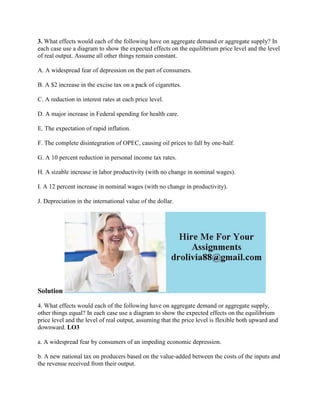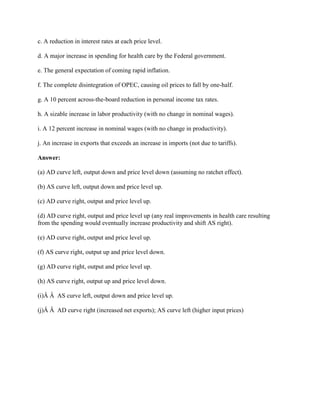Ce diaporama a bien été signalé.
Le téléchargement de votre SlideShare est en cours. ×

# 3- What effects would each of the following have on aggregate demand o.docx

Publicité
Publicité
Publicité
Publicité
Publicité
Publicité
Publicité
Publicité
Publicité
Publicité
PublicitéChargement dans…3
×

## Consultez-les par la suite

1 sur 3 Publicité

# 3- What effects would each of the following have on aggregate demand o.docx

3. What effects would each of the following have on aggregate demand or aggregate supply? In each case use a diagram to show the expected effects on the equilibrium price level and the level of real output. Assume all other things remain constant.
A. A widespread fear of depression on the part of consumers.
B. A \$2 increase in the excise tax on a pack of cigarettes.
C. A reduction in interest rates at each price level.
D. A major increase in Federal spending for health care.
E. The expectation of rapid inflation.
F. The complete disintegration of OPEC, causing oil prices to fall by one-half.
G. A 10 percent reduction in personal income tax rates.
H. A sizable increase in labor productivity (with no change in nominal wages).
I. A 12 percent increase in nominal wages (with no change in productivity).
J. Depreciation in the international value of the dollar.
Solution
4. What effects would each of the following have on aggregate demand or aggregate supply, other things equal? In each case use a diagram to show the expected effects on the equilibrium price level and the level of real output, assuming that the price level is flexible both upward and downward. LO3
a. A widespread fear by consumers of an impeding economic depression.
b. A new national tax on producers based on the value-added between the costs of the inputs and the revenue received from their output.
c. A reduction in interest rates at each price level.
d. A major increase in spending for health care by the Federal government.
e. The general expectation of coming rapid inflation.
f. The complete disintegration of OPEC, causing oil prices to fall by one-half.
g. A 10 percent across-the-board reduction in personal income tax rates.
h. A sizable increase in labor productivity (with no change in nominal wages).
i. A 12 percent increase in nominal wages (with no change in productivity).
j. An increase in exports that exceeds an increase in imports (not due to tariffs).
(a) AD curve left, output down and price level down (assuming no ratchet effect).
(b) AS curve left, output down and price level up.
(c) AD curve right, output and price level up.
(d) AD curve right, output and price level up (any real improvements in health care resulting from the spending would eventually increase productivity and shift AS right).
(e) AD curve right, output and price level up.
(f) AS curve right, output up and price level down.
(g) AD curve right, output and price level up.
(h) AS curve right, output up and price level down.
(i)Â Â  AS curve left, output down and price level up.
(j)Â Â  AD curve right (increased net exports); AS curve left (higher input prices)
.

3. What effects would each of the following have on aggregate demand or aggregate supply? In each case use a diagram to show the expected effects on the equilibrium price level and the level of real output. Assume all other things remain constant.
A. A widespread fear of depression on the part of consumers.
B. A \$2 increase in the excise tax on a pack of cigarettes.
C. A reduction in interest rates at each price level.
D. A major increase in Federal spending for health care.
E. The expectation of rapid inflation.
F. The complete disintegration of OPEC, causing oil prices to fall by one-half.
G. A 10 percent reduction in personal income tax rates.
H. A sizable increase in labor productivity (with no change in nominal wages).
I. A 12 percent increase in nominal wages (with no change in productivity).
J. Depreciation in the international value of the dollar.
Solution
4. What effects would each of the following have on aggregate demand or aggregate supply, other things equal? In each case use a diagram to show the expected effects on the equilibrium price level and the level of real output, assuming that the price level is flexible both upward and downward. LO3
a. A widespread fear by consumers of an impeding economic depression.
b. A new national tax on producers based on the value-added between the costs of the inputs and the revenue received from their output.
c. A reduction in interest rates at each price level.
d. A major increase in spending for health care by the Federal government.
e. The general expectation of coming rapid inflation.
f. The complete disintegration of OPEC, causing oil prices to fall by one-half.
g. A 10 percent across-the-board reduction in personal income tax rates.
h. A sizable increase in labor productivity (with no change in nominal wages).
i. A 12 percent increase in nominal wages (with no change in productivity).
j. An increase in exports that exceeds an increase in imports (not due to tariffs).
(a) AD curve left, output down and price level down (assuming no ratchet effect).
(b) AS curve left, output down and price level up.
(c) AD curve right, output and price level up.
(d) AD curve right, output and price level up (any real improvements in health care resulting from the spending would eventually increase productivity and shift AS right).
(e) AD curve right, output and price level up.
(f) AS curve right, output up and price level down.
(g) AD curve right, output and price level up.
(h) AS curve right, output up and price level down.
(i)Â Â  AS curve left, output down and price level up.
(j)Â Â  AD curve right (increased net exports); AS curve left (higher input prices)
.

Publicité
Publicité

### 3- What effects would each of the following have on aggregate demand o.docx

1. 1. 3. What effects would each of the following have on aggregate demand or aggregate supply? In each case use a diagram to show the expected effects on the equilibrium price level and the level of real output. Assume all other things remain constant. A. A widespread fear of depression on the part of consumers. B. A \$2 increase in the excise tax on a pack of cigarettes. C. A reduction in interest rates at each price level. D. A major increase in Federal spending for health care. E. The expectation of rapid inflation. F. The complete disintegration of OPEC, causing oil prices to fall by one-half. G. A 10 percent reduction in personal income tax rates. H. A sizable increase in labor productivity (with no change in nominal wages). I. A 12 percent increase in nominal wages (with no change in productivity). J. Depreciation in the international value of the dollar. Solution 4. What effects would each of the following have on aggregate demand or aggregate supply, other things equal? In each case use a diagram to show the expected effects on the equilibrium price level and the level of real output, assuming that the price level is flexible both upward and downward. LO3 a. A widespread fear by consumers of an impeding economic depression. b. A new national tax on producers based on the value-added between the costs of the inputs and the revenue received from their output.
2. 2. c. A reduction in interest rates at each price level. d. A major increase in spending for health care by the Federal government. e. The general expectation of coming rapid inflation. f. The complete disintegration of OPEC, causing oil prices to fall by one-half. g. A 10 percent across-the-board reduction in personal income tax rates. h. A sizable increase in labor productivity (with no change in nominal wages). i. A 12 percent increase in nominal wages (with no change in productivity). j. An increase in exports that exceeds an increase in imports (not due to tariffs). Answer: (a) AD curve left, output down and price level down (assuming no ratchet effect). (b) AS curve left, output down and price level up. (c) AD curve right, output and price level up. (d) AD curve right, output and price level up (any real improvements in health care resulting from the spending would eventually increase productivity and shift AS right). (e) AD curve right, output and price level up. (f) AS curve right, output up and price level down. (g) AD curve right, output and price level up. (h) AS curve right, output up and price level down. (i)Â Â AS curve left, output down and price level up. (j)Â Â AD curve right (increased net exports); AS curve left (higher input prices)